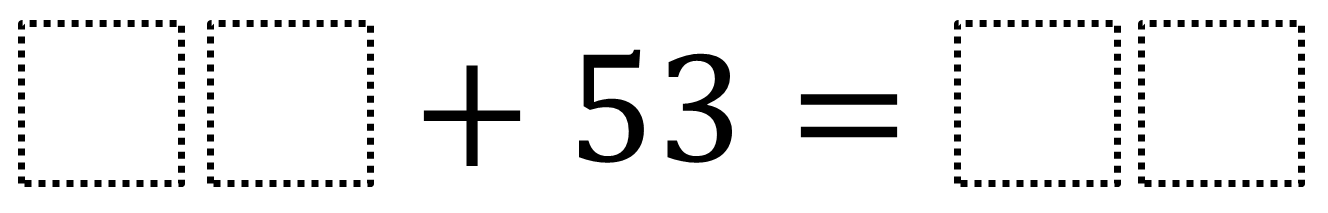Home > Grade 1 > Adding Two-Digit Numbers Given One

# Adding Two-Digit Numbers Given One

Directions: Fill in the boxes below using the whole numbers 0 through 9, at most one time each, so that you can make a true equation.### Hint

How can we ensure that all numbers will be 2-digits?

There are many answers including 27 + 53 = 80. Wrong answers include 76 + 53 = 129 because the sum needs to be two-digits.

Source: Robert Kaplinsky

## Adding with Parenthesis

Directions: Using the digits 1 to 9, at most one time each, make a true …

1.Christine Roberts

I am thinking that the digits should be changed to include 0-9 in order to have a solution such as 27 + 53 = 80 (sample answer). If you want to use only the digits 1-9, then a different sample answer should be provided such as 26 + 53 = 79.

•You are totally right, Christine. I changed it to 0-9.

•Hi, maybe the directions should say to use any of the numbers between 1-9, some will not be used

•Yeah, that would certainly need to get cleared up while introducing the problem to students.

2.Should 5 and 3 be excluded since they are already in the problem?

3.My 1st graders came up with a number of solutions. 12 + 53 = 65 , 26 + 53 = 79 , 20 + 53 = 73, 10 + 53 = 63 , and 41 + 53 = 63. Afterwards, the class tried to find the lowest/highest sum.